# How to Calculate and Solve for the Area, Width and Length of a Rectangle | The Calculator Encyclopedia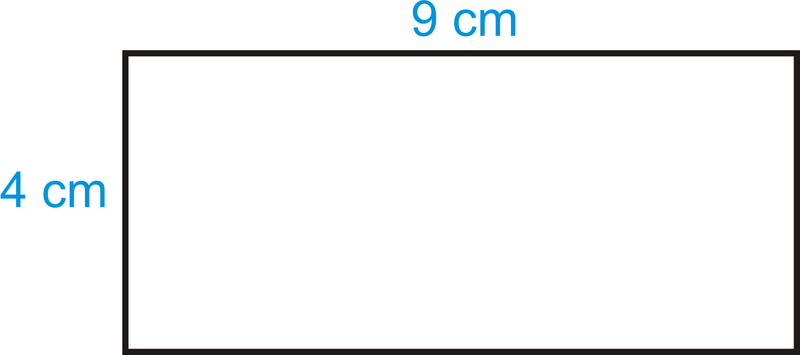The image above is a rectangle with a length of 9 cm and a width of 4 cm.
To compute the area of a rectangle requires two essential parameters which are the length of the rectangle and the width of the rectangle.

The formula for calculating the area of a rectangle is:

A = lw

Where:

A = Area of a Rectangle
l = Length of a Rectangle
w = Width of a Rectangle

Let’s solve an example
Find the area of a rectangle where the length of the rectangle is 9 cm and the width of the rectangle is 4 cm.

This implies that:
l = Length of the Rectangle = 9 cm
w = Width of the Rectangle = 4 cm

A = lw
A = (9)(4)
A = 36

Therefore, the area of the rectangle is 36 cm2.

How to Calculate the Length of a Rectangle when the Area and Width of the Rectangle is Given

The formula for calculating the length of the rectangle is:

l = A / w

Where:

l = Length of the Rectangle
A = Area of the Rectangle
w = Width of the Rectangle

Let’s solve an example:
Find the length of a rectangle where the area of the rectangle is 45 cm2 and the width of the rectangle is 9 cm.

This implies that:
A = Area of the Rectangle = 45 cm2
w = Width of the Rectangle = 9 cm

l = A / w
l = 45 / 9
l = 5

Therefore, the length of the rectangle is 5 cm.

How to Calculate the Width of a Rectangle when the Area and Length of the Rectangle is Given

The formula for calculating the width of the rectangle is:

w = A / l

Where:

w = Width of the Rectangle
A = Area of the Rectangle
l = Length of the Rectangle

Let’s solve an example:
Find the width of a rectangle where the area of the rectangle is 60 cm2 and the length of the rectangle is 10 cm.

This implies that:
A = Area of the Rectangle is 60 cm2
l = Length of the Rectangle is 10 cm

w = A / l
w = 60 / 10
w = 6

Therefore, the width of the rectangle is 6 cm.

Nickzom Calculator – The Calculator Encyclopedia is capable of calculating the area of a rectangle.

To get the answer and workings of the area of a rectangle using the Nickzom Calculator – The Calculator Encyclopedia. First, you need to obtain the app.

You can get this app via any of these means:

To get access to the professional version via web, you need to register and subscribe for NGN 1,500 per annum to have utter access to all functionalities.
You can also try the demo version via https://www.nickzom.org/calculator

Once, you have obtained the calculator encyclopedia app, proceed to the Calculator Map, then click on Mensuration under the Mathematics section.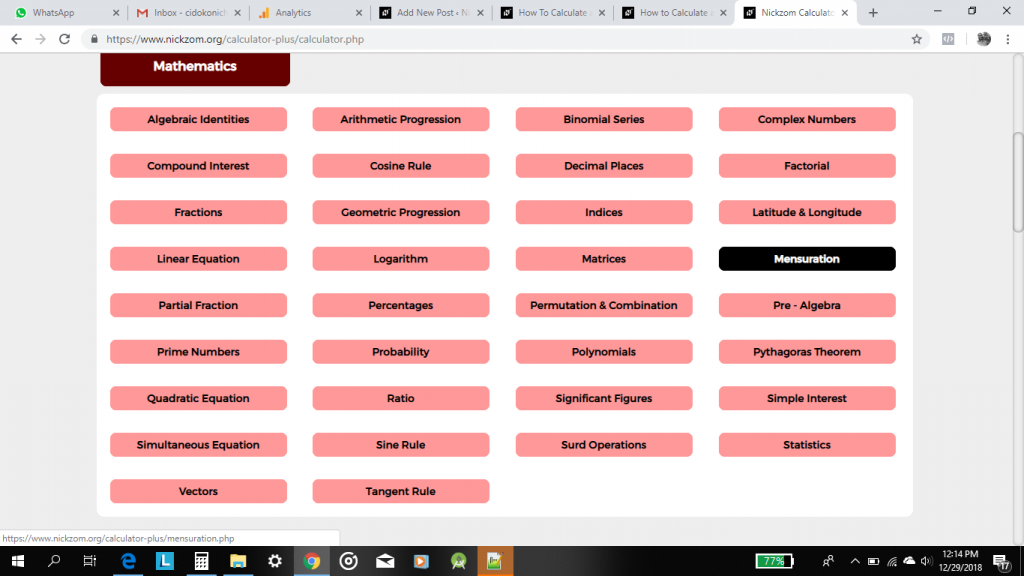Now, click on Area of a Rectangle under Mensuration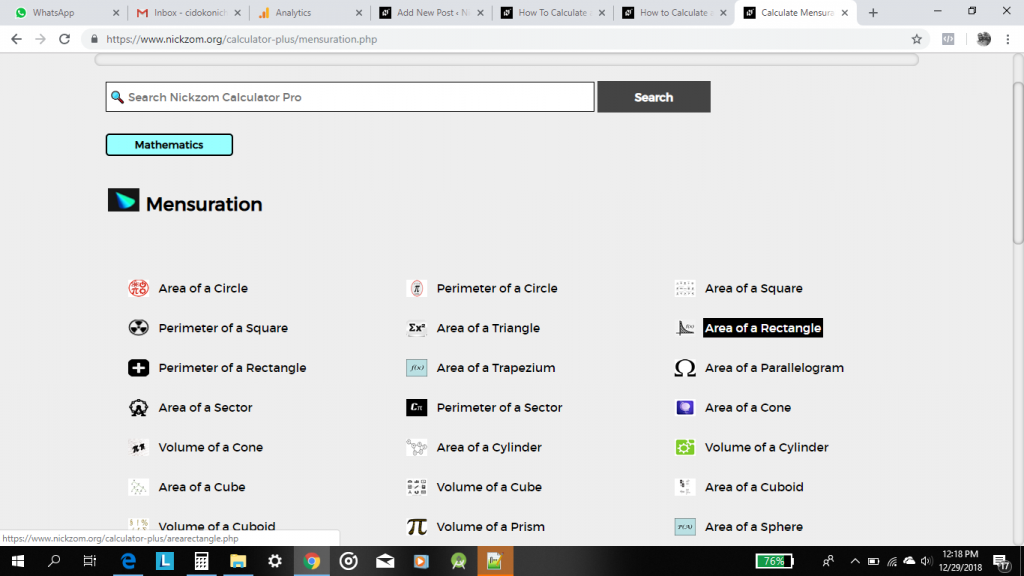The screenshot below displays the page or activity to enter your values, to get the answer for the area of a rectangle according to the respective parameters which are the length of the rectangle (l) and the width of the rectangle (w).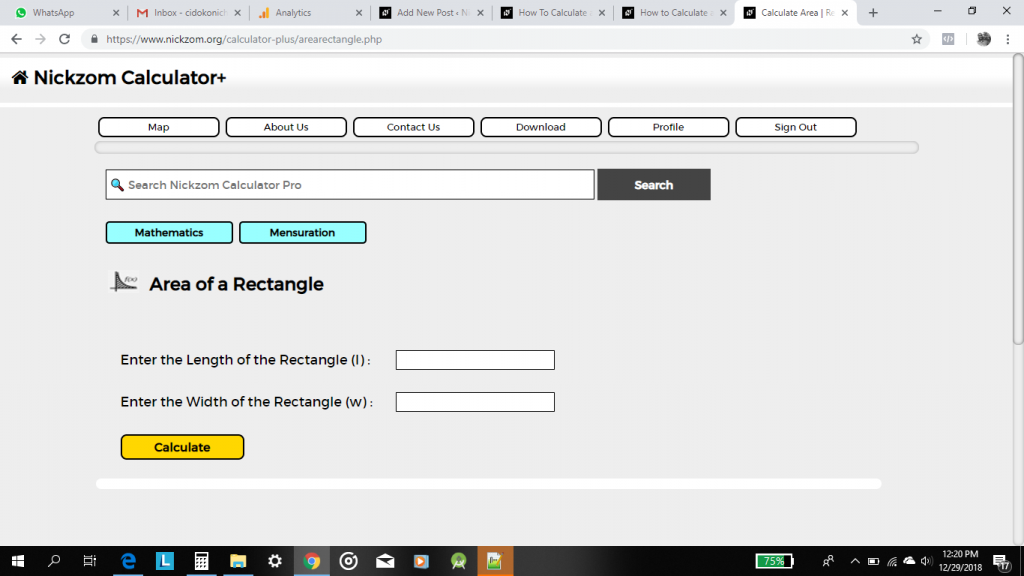Now, enter the values appropriately and accordingly for the parameters as required by the example above where the length of the rectangle is 9 cm and the width of the rectangle is 4 cm.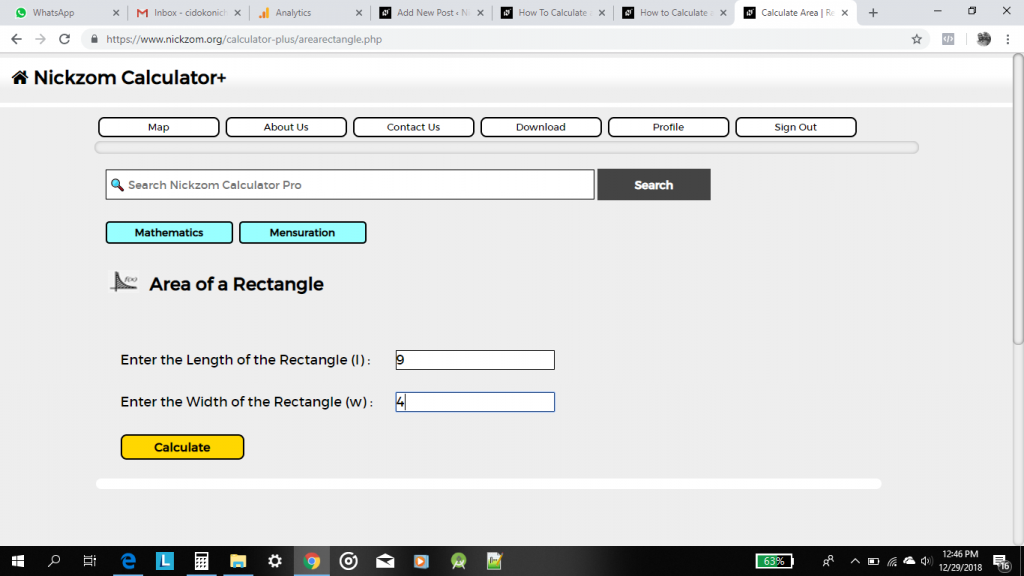Finally, click on Calculate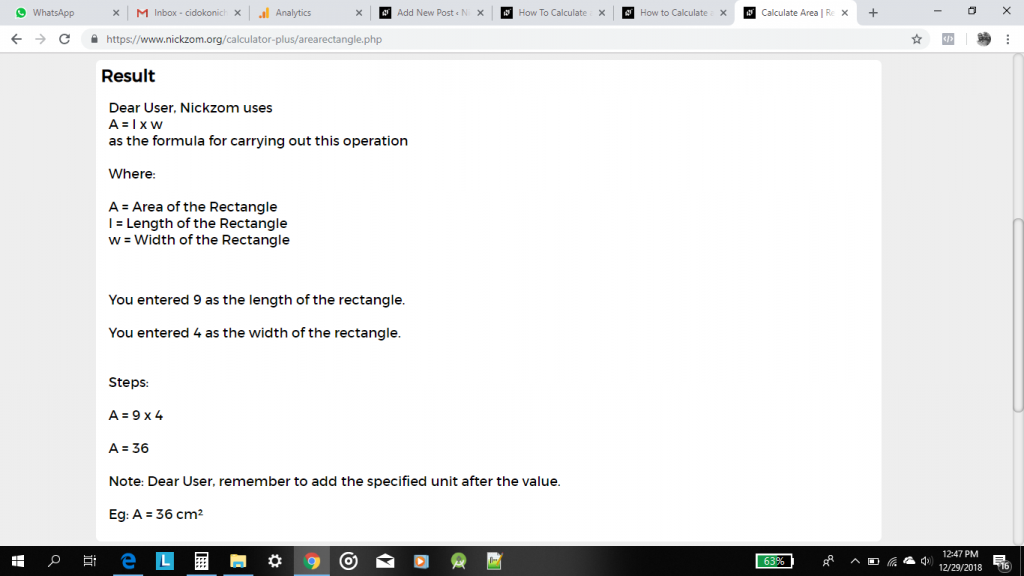As you can see from the screenshot above, Nickzom Calculator – The Calculator Encyclopedia solves for the area of a rectangle and presents the formula, workings and steps too.